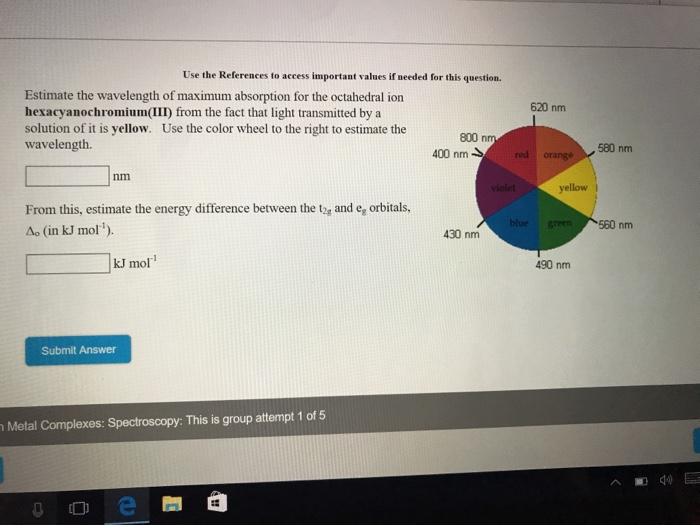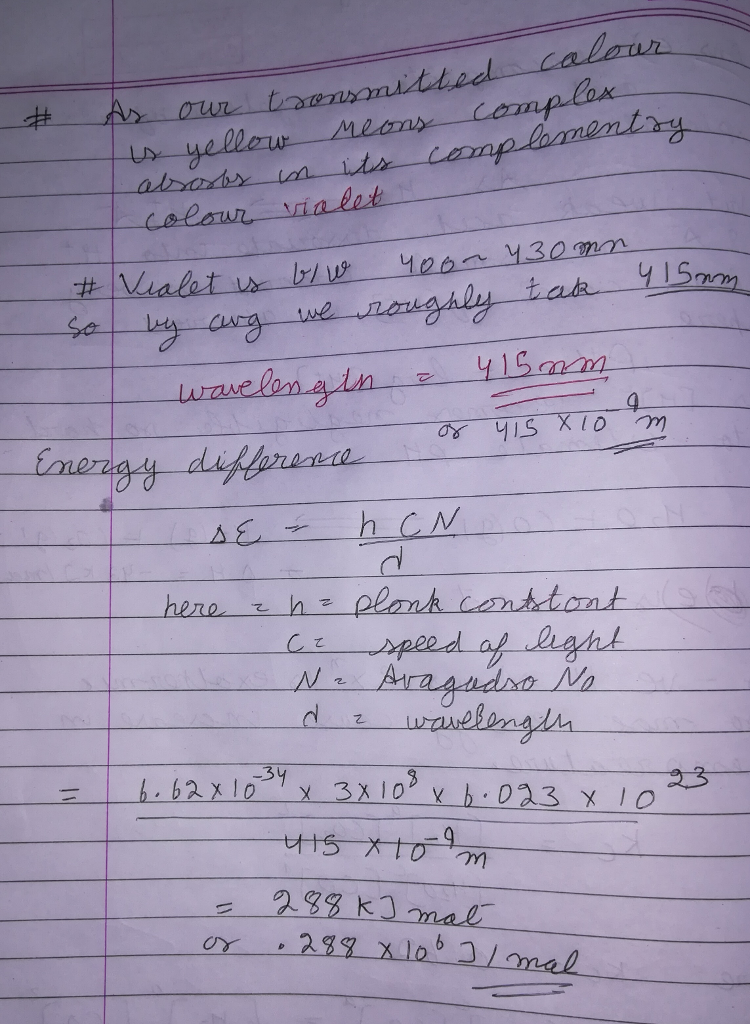# Question & Answer: Use the References to access important values if needed for this question. Estimate the…..Use the References to access important values if needed for this question. Estimate the wavelength of maximum absorption for the octahedral ion hexacyanochromium(III) from the fact that light transmitted by a solution of it is yellow. Use the color wheel to the right to estimate the wavelength. nm From this, estimate the energy difference between the t_2g and e_g orbitals, Delta_o (in kJ mol^-1). KJ mol^-1